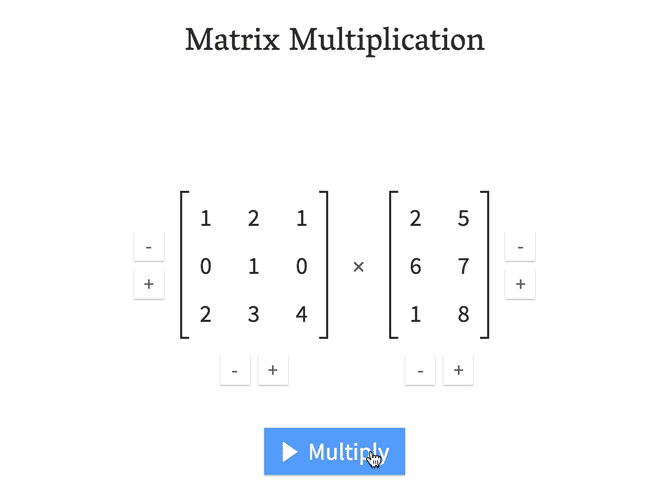# Matrix Waterfall Visualization of Dot Product

I found this interesting website where a person described how they realized how a dot product simulates a waterfall of the two matrices being combined.As he stated it:

When I first learned about matrix multiplication in high school, it wasn't easy to memorize the method, and it didn't make sense. It felt like someone had invented a weird way of blending those numbers together. Why was it so natural and logical to multiply scalar numbers, but not so when it comes to matrices of numbers?

This question bothered me a few times until I studied math in the university. There, I had in total four linear algebra courses, so matrix multiplication became my bread-and-butter. One day it just snapped in my mind how the number of rows of the first matrix has to match the number of columns in the second matrix, which means they must perfectly align when the second matrix is rotated by 90°. From there, the second matrix trickles down, "combing" the values in the first matrix. The values are multiplied and added together. In my head, I called this the "waterfall method", and used it to perform my calculations in the university courses. It worked.

To this day, I'm not sure if this method has been discovered by others and taught in schools and universities. I haven't found anything similar on the web, at least. So I decided to build this matrix multiplication calculator to help visualize the waterfall method. I hope it will help clarify matrix multiplication for high school and college students, who like me, don't like to memorize arbitrary methods, but prefer self-evident mnemonics.

http://matrixmultiplication.xyz/